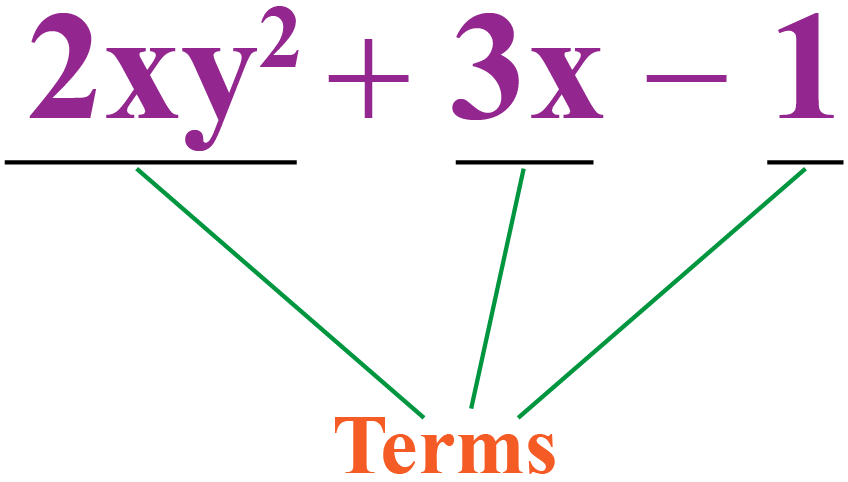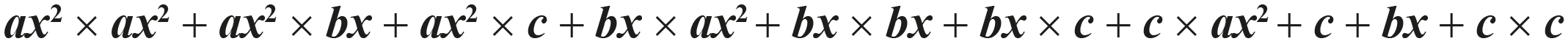# Squaring a Trinomial

Squaring a Trinomial

We have learned about numbers and their squares.

For example, if you were asked to find the square of 8, you would immediately say 64 because 8 x 8 = 64.

However, what answer would you give if someone asked you to square a polynomial

You will multiply the polynomial by itself.

Let us take the example of

$(x + y)^2$.

This becomes:

$(x+y)\times(x+y)$

$\therefore x^2 + 2xy + y^2$

In this mini-lesson, we will explore how we can square a trinomial, which is a type of polynomial.

## Lesson Plan

 1 What Is a Trinomial? 2 Important Notes on Squaring a Trinomial 3 Solved Examples on Squaring a Trinomial 4 Challenging Questions on Squaring a Trinomial 5 Interactive Questions on Squaring a Trinomial

## What Is a Trinomial?

Before learning about trinomials,  let us first understand what a polynomial is.

### Polynomial

A polynomial is a mathematical expression written in the form:

$a_0x^n + a_1x^{n-1} + a_2x^{n-2}...........+ a_nx^0$

The above expression is also called polynomials in the standard form.

Where $$a_0, a_1, a_2.........a_n$$ are constants and $$n$$ is a natural number.

### Trinomial

A trinomial meaning in math is, it is a type of polynomial that contains only three terms.The expressions $$x^2 + 2x + 3$$,  $$5x^4 - 4x^2 +1$$ and $$7y - \sqrt{3} - y^2$$ are trinomial examples.

The above trinomial examples are the examples with one variable only, let's take a few more trinomial examples with multiple variables.

$$x^2 + y^2 + xy$$,  $$5x^4 - 4x^2 + z$$ and $$xyz^3 + x^2z^2 + zy^3$$ are trinomials with multiple variables.

Let's recall the names used to refer to polynomials with a different number of terms.

 Number of Terms Example Polynomial 1 $$xy$$ Monomial 2 $$x + y$$ Binomial 3 $$x^2 + xz + 1$$ Trinomial

Polynomials with more than 3 terms are simply referred to as "Polynomials".

There are no such special names for these types of polynomials.

## How to Square a Trinomial?

Let's take a general trinomial in one variable.

$P(x) = ax^2 + bx + c$

Now, if we square a trinomial it means that we are squaring $$P(x)$$.

${P(x)}^2$

${(ax^2 + bx + c)}^2$

The above expression means that we are multiplying the trinomial to itself once.

${(ax^2 + bx + c)}^2 = (ax^2 + bx + c) \times (ax^2 + bx + c)$

Now, multiply each term of the first trinomial to the second trinomial.

$${(ax^2 + bx + c)}^2$$, on expanding this expression can be written as:${a^2}{x^4} + abx^3 + acx^2 + abx^3 + {b^2}{x^2} + bcx + acx^2 + bcx + c^2$

Combine the like terms.

${a^2}{x^4} + (abx^3 + abx^3) + (acx^2 + acx^2 + {b^2}{x^2}) + (bcx + bcx) + c^2$

${a^2}{x^4} + 2abx^3 + (2acx^2 + {b^2}{x^2}) + 2bcx + c^2$

${a^2}{x^4} + 2abx^3 + (2ac + b^2)x^2 + 2bcx + c^2$

The above result can be refferd as the squaring a trinomial formula.

Let's take one example of squaring a trinomial.

Example

Find the square of the trinomial $$P(x) = x^2 + 2x + 3$$

Solution

If we compare the above trinomial with a general trinomial in one variable we can say that:

$$a = 1, b = 2, c = 3$$

So, $${(x^2 + 2x + 3)}^2$$ can be calculated by putting the values of $$a, b, c$$ in the result of squaring a trinomial.

${a^2}{x^4} + 2abx^3 + (2ac + b^2)x^2 + 2bcx + c^2$

${1^2}{\times}{x^4} + 2{\times}1{\times}2{\times}x^3 + (2{\times}1{\times}3 + 2^2)x^2 + 2{\times}2{\times}3{\times}x + 3^2$

$x^4 + 4x^3 + (6 + 4)x^2 + 12{\times}x + 9$

$x^4 + 4x^3 + 10x^2 + 12x + 9$

We usually do no prefer to remember this big formula, so for finding the square of any trinomial we prefer using the actual multiplication of two trinomials.

Let's take one more example of squaring a trinomial.

Example

Find the square of the trinomial $$P(x) = xy + x + 1$$

Solution

$${(xy + x + 1)}^2$$ can be calculated by multiplying the polynomial to itself twice.

${(xy + x + 1)}^2 = (xy + x + 1){\times}(xy + x + 1)$

$xy(xy + x + 1) + x(xy + x + 1) + 1(xy + x + 1)$

$xy{\times}xy + xy{\times}x + xy{\times}1 + x{\times}xy + x{\times}x + x{\times}1 + 1{\times}xy + 1{\times}x + 1{\times}1$

${x^2}{y^2} + {x^2}y + xy + {x^2}y + x^2 + x + xy + x + 1$

${x^2}{y^2} + 2{x^2}y + 2xy + x^2 + 2x + 1$

${x^2}{y^2} + (2y + 1){x^2} + 2xy + 2x + 1$

## Squaring a Trinomial Calculator

We have learn how to square a trinomial by multiplying a trinomial to itself, let's now explore the squaring a trinomial calculator to understand the squaring of trinimials into more detail.

Enter a trinomial in the simulation below to find out its square.

## Solved Examples on Squaring a Trinomial

 Example 1

Help Tom to find the square of the trinomial $$x + y + z$$.

Solution

The square of the trinomial $$x + y + z$$ is $${(x + y + z)}^2$$

\begin{align}{(x + y + z)}^2 &= (x + y + z)(x + y + z) \\[0.2cm] &= x(x + y + z) + y(x + y + z) + z(x + y + z) \\[0.2cm] &= (x^2 + xy + xz) + (xy + y^2 + yz) + (xz + yz + z^2) \\[0.2cm] &= x^2 + y^2 + z^2 + (xy + xy) + (yz + yz) + (xz + xz) \\[0.2cm] &= x^2 + y^2 + z^2 + 2xy + 2yz + 2xz \\[0.2cm] &= x^2 + y^2 + z^2 + 2(xy + yz + xz) \end{align}

 $$\therefore$$ $$x^2 + y^2 + z^2 + 2(xy + yz + xz)$$
 Example 2

Paul says that the coefficient of $$xy$$ in the square of the trinomial $$x^2y + x + y$$ is 1. Is he right?

Solution

To find out whether Paul is right or not let's square the trinomial $$x^2y + x + y$$

The square of the trinomial $$x^2y + x + y$$ is $${(x^2y + x + y)}^2$$

\begin{align}{({x^2}y + x + y)}^2 &= (x^2y + x + y)(x^2y + x + y) \\[0.2cm] &= x^2y(x^2y + x + y) + x(x^2y + x + y) + y(x^2y + x + y) \\[0.2cm] &= (x^4y^2 + x^3y + x^2y^2) + (x^3y + x^2 + xy) + (x^2y^2 + xy + y^2) \\[0.2cm] &= x^4y^2 + (x^3y + x^3y) + (x^2y^2 + x^2y^2) + x^2 + y^2 + (xy + xy) \\[0.2cm] &= x^4y^2 + 2x^3y + 2x^2y^2 + x^2 + y^2 + 2xy \\[0.2cm] &= x^4y^2 + x^2 + y^2 + 2x^3y + 2x^2y^2 + 2xy \end{align}

Coefficient of $$xy$$ in the square of the trinomial $$x^2y + x + y$$ is 2

 $$\therefore$$ Paul is incorrect.Challenging Questions
• Find the coefficent of $$x^4$$ in the expansion of $${(x + 1)}^6$$.
• Find the square root of the trinomial $$81x^2 + 126xy + 49y^2$$.

## Let's Summarize

The mini-lesson targeted the fascinating concept of squaring a trinomial. The math journey around squaring a trinomial starts with what a student already knows, and goes on to creatively crafting a fresh concept in the young minds. Done in a way that is not only relatable and easy to grasp, but will also stay with them forever. Here lies the magic with Cuemath.

At Cuemath, our team of math experts is dedicated to making learning fun for our favorite readers, the students!

Through an interactive and engaging learning-teaching-learning approach, the teachers explore all angles of a topic.

Be it worksheets, online classes, doubt sessions, or any other form of relation, it’s the logical thinking and smart learning approach that we, at Cuemath, believe in.

### 1. What is a trinomial in math?

A trinomial meaning in math is, it is a type of polynomial that contains only three terms.
For example, $$x - 2y - z$$ or $$x^2 + y^2 + xy$$

### 2. What is a trinomial square?

Let's take a trinomial $$P(x) = x + y + xy$$.
So, a trinomial square is the square of $$P(x)$$.
$${P(x)}^2$$ is the trinomial square for $$P(x)$$.
$${P(x)}^2 = {(x + y + xy)}^2 = (x + y + xy)(x + y + xy)$$

### 3.Which polynomial is a perfect square trinomial?

Polynomials like $$x^2 + 2x + 1$$ is an example of perfect square trinomial.
$$x^2 + 2x + 1$$ can be written as $${(x + 1)}^2$$.

### 4. How do you square a trinomial?

A trinomial can be squared by multiplying itself twice and performed the required calculations.
Explore the calculator above to find out the squaring of a trinomial in more detail.